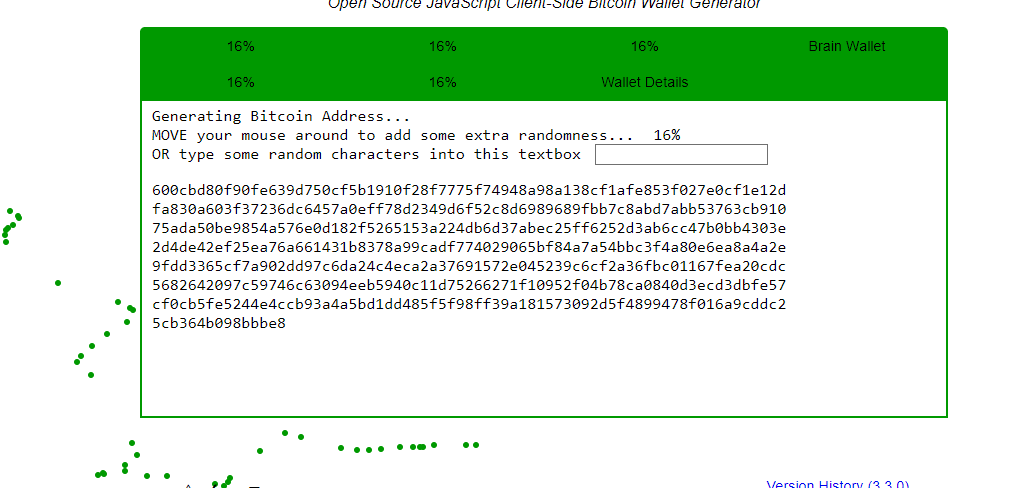## 引介 | 以太坊 2.0：随机性## 计算机中的随机性## 计算机是无法生成真正的随机数的。## 区块链中的随机性## 简单概述 RANDAORANDAO 的本质就是如此。RANDAO 机制就是，当用户通过储存（质押）32 ETH 成为验证者之后，该用户可以任意选定一个随机数。当需要为某个区块公布随机数时，将所有验证者的随机数加起来就可以得到一个全新的随机数。

## RANDAO + VDF

VDF 全称为 Verifiable Delay Function（可验证延迟函数)。

``((((((X^2)^2)^2)^2)^2)^2)^2``

```

`((((((5^2)^2)^2)^2)^2)^2)^2 =``(((((25^2)^2)^2)^2)^2)^2 =``((((625^2)^2)^2)^2)^2 =``(((390625^2)^2)^2)^2 =``((152587890625^2)^2)^2 =``(23283064365386962890625^2)^2 =``542101086242752217003726400434970855712890625^2 =``293873587705571876992184134305561419454666389193021880377187926569604314863681793212890625`Nimbus 中的随机性https://our.status.im/two-point-oh-randomness/

```